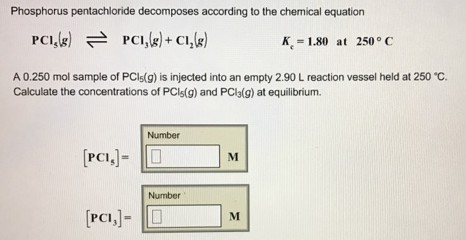# Problem: Phosphorus pentachloride decomposes according to the chemical equation PCI5(g) ⇌ PCI3(g) + Cl2(g)         Kc = 1.80 at 250°C A 0.250 mol sample of PCI5(g) is injected into an empty 2.90 L reaction vessel held at 250°C. Calculate the concentrations of PCl5(g) and PCl3(g) at equilibrium. [PCl5] = [PCl3] =

###### FREE Expert Solution
81% (182 ratings)###### Problem Details

Phosphorus pentachloride decomposes according to the chemical equation

PCI5(g) ⇌ PCI3(g) + Cl2(g)         Kc = 1.80 at 250°C

A 0.250 mol sample of PCI5(g) is injected into an empty 2.90 L reaction vessel held at 250°C. Calculate the concentrations of PCl5(g) and PCl3(g) at equilibrium.

[PCl5] =

[PCl3] =